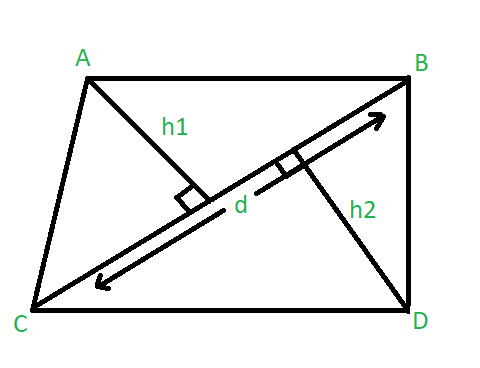# Find the area of quadrilateral when diagonal and the perpendiculars to it from opposite vertices are given

• Last Updated : 22 Mar, 2021

Given three integers d, h1, h2 where d represents the length of the diagonal of a quadrilateral. h1 and h2 represents the lengths of the perpendiculars to the given diagonal from the opposite vertices. The task is to find the area of the Quadrilateral.Examples:

Input : d= 6, h1 = 4, h2 = 3
Output : 21
Input : d= 10, h1 = 8, h2 = 10
Output : 90

Approach :
Area of the quadrilateral is the sum of the areas of both triangles. We know that the area of the triangle is 1/2*base*height.
Therefore, the area of a quadrilateral can be calculated as :

Area = 1/2 * d * h1 + 1/2 * d * h2
= 1/2 * d * ( h1 + h2 )

Below is the implementation of the above approach :

## C++

 `// C++ program to find the area of quadrilateral``#include ``using` `namespace` `std;` `// Function to find the area of quadrilateral``float` `Area(``int` `d, ``int` `h1, ``int` `h2)``{``    ``float` `area;` `    ``area = 0.5 * d * (h1 + h2);` `    ``return` `area;``}` `// Driver code``int` `main()``{``    ``int` `d = 6, h1 = 4, h2 = 3;` `    ``cout << ``"Area of Quadrilateral = "` `<< (Area(d, h1, h2));` `    ``return` `0;``}`

## Java

 `// Java program to find the area of quadrilateral``class` `GFG``{` `    ``// Function to find the area of quadrilateral``    ``static` `float` `Area(``int` `d, ``int` `h1, ``int` `h2)``    ``{``        ``float` `area;` `        ``area = (``float``) ``0.5` `* d * (h1 + h2);` `        ``return` `area;``    ``}` `    ``// Driver code``    ``public` `static` `void` `main(String[] args)``    ``{``        ``int` `d = ``6``, h1 = ``4``, h2 = ``3``;` `        ``System.out.println(``"Area of Quadrilateral = "` `+``                                      ``Area(d, h1, h2));``    ``}``}` `// This code is contributed by Princi Singh`

## Python3

 `# Python3 program to find``# the area of quadrilateral` `# Function to find the``# area of quadrilateral``def` `Area(d, h1, h2):` `    ``area ``=` `0.5` `*` `d ``*` `(h1 ``+` `h2);` `    ``return` `area;` `# Driver code``if` `__name__ ``=``=` `'__main__'``:``    ` `    ``d ``=` `6``;``    ``h1 ``=` `4``;``    ``h2 ``=` `3``;` `    ``print``(``"Area of Quadrilateral = "``,``                  ``(Area(d, h1, h2)));` `# This code is contributed by Rajput-Ji`

## C#

 `// C# program to find the area of quadrilateral``using` `System;` `class` `GFG``{``    ` `// Function to find the area of quadrilateral``static` `float` `Area(``int` `d, ``int` `h1, ``int` `h2)``{``    ``float` `area;` `    ``area = (``float``)0.5 * d * (h1 + h2);` `    ``return` `area;``}`  `// Driver code``public` `static` `void` `Main()``{``    ``int` `d = 6, h1 = 4, h2 = 3;``    ` `    ``Console.WriteLine(``"Area of Quadrilateral = "` `+``                                 ``Area(d, h1, h2));``}``}` `// This code is contributed by nidhiva`

## Javascript

 ``
Output:
`Area of Quadrilateral = 21`

My Personal Notes arrow_drop_up